• #include using namespace std; int FreePartition;...//首次适应算法数组 int CycleFirstPartition;//循环首次适应算法数组 int BestPartition;//最佳适应算法数组 int WorstPartiti
#include<iostream>
using namespace std;

int FreePartition;//空闲分区块数组
int FirstPartition;//首次适应算法数组
int CycleFirstPartition;//循环首次适应算法数组
int BestPartition;//最佳适应算法数组
int WorstPartition;//最坏适应算法数组
int ProcessNeed;//每个作业的大小
int PartitionNum,ProcessNum;//分区块数，作业数

//首次适应算法
void First()
{
int i,j;
char str;
for(i=0;i<PartitionNum;i++)
{
FirstPartition[i]=FreePartition[i];
}
for(i=0;i<ProcessNum;i++)//找出第一块满足作业的分区
for(j=0;j<PartitionNum;j++)
{
if(ProcessNeed[i]>FirstPartition[j])
continue;
else
{
FirstPartition[j]-=ProcessNeed[i];//找到后把分区大小减去作业的大小
str='A'+i;
cout<<"作业"<<str<<"在第"<<j+1<<"块分区中"<<endl;
break;
}
}
cout<<endl;
cout<<"分配之后剩余情况："<<endl;
for(i=0;i<PartitionNum;i++)
cout<<FirstPartition[i]<<" ";
cout<<endl<<endl;
}

//循环首次适应算法
void CycleFirst()
{
int i,j=1;
char str;
for(i=0;i<PartitionNum;i++)
{
CycleFirstPartition[i]=FreePartition[i];
}
for(i=0;i<ProcessNum;i++)
//for(j=0;j<PartitionNum;j++)
{
j=j-1;
while(j<PartitionNum)
{
if(ProcessNeed[i]>CycleFirstPartition[j])
//continue;
j++;
else
{
CycleFirstPartition[j]-=ProcessNeed[i];
str='A'+i;
cout<<"作业"<<str<<"在第"<<j+1<<"块分区中"<<endl;
break;
}
//j++;
//cout<<j<<" ";
if(j==PartitionNum && i!=ProcessNum)
{
i=-1;
}
}
}
cout<<endl;
cout<<"分配之后剩余情况："<<endl;
for(i=0;i<PartitionNum;i++)
cout<<CycleFirstPartition[i]<<" ";
cout<<endl<<endl;

}

//最佳适应算法
void Best()
{
int i,j,k;
char str;
for(i=0;i<PartitionNum;i++)
{
BestPartition[i]=FreePartition[i];
}
for(i=0;i<ProcessNum;i++)
{
k=0;
for(j=0;j<PartitionNum;j++)
{
//cout<<BestPartition[j]<<"   "<<ProcessNeed[i]<<endl;
if(BestPartition[j]>=ProcessNeed[i])
{
k=j;
break;
}
}
for(int n=0;n<PartitionNum;n++)
{
if(BestPartition[n]<BestPartition[k] && BestPartition[n]>=ProcessNeed[i])//找最佳的
k=n;
}
BestPartition[k]-=ProcessNeed[i];
str='A'+i;
cout<<"作业"<<str<<"在第"<<j+1<<"块分区中"<<endl;
}
cout<<endl;
cout<<"分配之后剩余情况："<<endl;
for(i=0;i<PartitionNum;i++)
cout<<BestPartition[i]<<" ";
cout<<endl<<endl;

}

//最坏适应算法
void Worst()
{
int i,j,k;
char str;
for(i=0;i<PartitionNum;i++)
{
WorstPartition[i]=FreePartition[i];
}
for(i=0;i<ProcessNum;i++)
{
k=0;
for(j=0;j<PartitionNum;j++)
{
if(WorstPartition[j]>WorstPartition[k])//找到最大的分区
k=j;
}
WorstPartition[k]-=ProcessNeed[i];
str='A'+i;
cout<<"作业"<<str<<"在第"<<j+1<<"块分区中"<<endl;
}
cout<<endl;
cout<<"分配之后剩余情况："<<endl;
for(i=0;i<PartitionNum;i++)
cout<<WorstPartition[i]<<" ";
cout<<endl<<endl;
}

int main()
{
int i;
cout<<"输入分区块数："<<endl;
cin>>PartitionNum;
cout<<"输入每个分区的大小："<<endl;
for(i=0;i<PartitionNum;i++)
cin>>FreePartition[i];
cout<<"输入作业数："<<endl;
cin>>ProcessNum;
cout<<"输入每个作业的大小："<<endl;
for(i=0;i<ProcessNum;i++)
cin>>ProcessNeed[i];
cout<<"------------首次适应算法-----------------"<<endl;
First();
cout<<"------------循环首次适应算法-------------"<<endl;
CycleFirst();
cout<<"------------最佳适应算法-----------------"<<endl;
Best();
cout<<"------------最坏适应算法-----------------"<<endl;
Worst();
return 0;
}

展开全文• 操作系统中利用最佳适应算法 最坏适应算法 循环首次适应算法 首次适应算法实现动态内存的分配回收内存
• 实验报告 班级： 计算机B191 学号： 2019537130 姓名： 陈力源 日期： 5月19日 ⒈ 实验题目 主存储器空间分配实验。...能分别使用首次适应算法...通过首次适应算法、最佳适应算法和最坏适应算法实现主存空间的...
实验报告

⒈ 实验题目

主存储器空间分配实验。

2．实验要求

编写一段程序来模拟可变分区管理方法。要求能通过文件形式定义空闲区表；能随意输入作业及需要分配的空间；能分别使用首次适应算法、最佳适应算法和最坏适应算法对输入的作业进行空间分配；能显示系统空闲表和已分配空间表。

3. 实验目的

通过首次适应算法、最佳适应算法和最坏适应算法实现主存空间的分配，可以使读者可好地理解存储分配算法。

⒋ 实验原理分析

⑴可变分区方式是按作业需要的主存空间大小来分区。当装入一个作业时，首先要查看是否有足够的空闲空间来分配，若有则按指定的分配方式进行分配；否则作业不能装入。随着作业的装入和撤离主存空间被分为若干个大大小小的不连续的区间，为了表明各区间的状态可以用一个内存分区表如表1所示来表示。

表1  内存分区表

起始地址

长度

标志

120k

20k

作业1

200k

50k

空闲

这样我们可以定义一个如下的结构表示内存分区信息。

typedef struct node

{

int start;                    //起始地址

int length;              //长度

char tag;             //标志

}job;

⑵可变分区的三种算法就是为作业分配主存空间的方法。

● 首次适应算法：在空闲区间中查询满足作业需要的空间，并将作业装入第一个满足条件的空间中去。

● 最佳适应算法：在空闲区间中查询满足作业需要的空间，并将作业装入满足条件的空闲空间中最小的一个空间中去。

● 最坏适应算法：在空闲区间中查询满足作业需要的空间，并将作业装入满足条件的空闲空间中最大的一个空间中去。

从三种算法的说明可以看出，分配空间的过程主要可以分两步：

● 查询所有满足作业需求的空间块。

● 按照指定的算法将作业装入空间块中。

⑶在操作的最初主存空间实际就是一个大的空闲区，不涉及到如何分配的问题。为直接模拟运行一段时间后主存中出现了多个空闲块的状态，题目要求从一个文件读入空闲区表。在这里我们可以设计一个空闲区表文件的结构为如表2所示：

表2  空闲区表

起始地址

长度

200k

50k

…

…

这样也可以方便地将空闲表一次读入程序中，而不必再一个个的输入。

⑷主要变量及函数说明如表3所示。

表3  变量与函数说明表

typedef struct node

内存块结构

job frees

空闲区表

job occupys

已分配区表

free_quantity

空闲区数量

occupy_quantity

已分配区数量

void initial()

初始化函数

int readData()

从文件读入空闲表函数

void sort()

排序空闲表

void view()

显示分区信息

void earliest()

最先适应分配算法

void excellent()

最优适应分配算法

void worst()

最坏适应算法

mem.txt

空闲表文件

5.实验代码清单

#include "stdafx.h"

#include "stdafx.h"

#include<stdio.h>

#include<stdlib.h>

#include<string.h>

#define MAXJOB 200 // 定义存储数据最大值

typedef struct node // 内存块结构

{

int start;

int length;

char tag;

}job;

job frees[MAXJOB]; // 定义空闲区表

int free_quantity; // 空闲区块数

job occupys[MAXJOB]; // 定义已分配区表

int occupy_quantity; // 已分配区块数

// 初始化函数

void initial()

{

int i;

for (i = 0; i < MAXJOB; i++)

{

frees[i].start = -1;

frees[i].length = 0;

strcpy(frees[i].tag, "free"); // 定义所有块为空闲块

occupys[i].start = -1;

occupys[i].length = 0;

strcpy(occupys[i].tag, ""); // 已分配块为 0

}

free_quantity = 0;

occupy_quantity = 0;

}

// 读数据函数，从文件中读入空闲表的设置

int readData()

{

FILE * fp;

if ((fp = fopen("D:\\mem.txt", "r")) == NULL)

{

printf(" 错误, 文件不存在\n");

}

else

{

while (!feof(fp))

{ // 打开文件读入空闲表信息

fscanf(fp, "%d %d", & frees[free_quantity].start, & frees[free_quantity].length);

free_quantity++;

}

return 1;

}

return 0;

}

// 排序空闲表

void sort()

{

int i, j, p;

for (i = 0; i < free_quantity - 1; i++)

{

p = i;

for (j = i + 1; j < free_quantity; j++)

{

if (frees[j].start < frees[p].start)

{

p = j;

}

}

if (p != i)

{

frees[free_quantity] = frees[i];

frees[i] = frees[p];

frees[p] = frees[free_quantity];

}

}

}

// 显示函数

void view()

{

int i;

printf(" 空闲表 :\n"); // 显示空闲表

printf(" 起始地址   长度  状态\n");

for (i = 0; i < free_quantity; i++)

{

printf("%8d\t%4d\t%s\n", frees[i].start, frees[i].length, frees[i].tag);

}

getchar();

}

void prin()

{

printf(" 当前已分配表 :\n");

printf(" 起始地址   长度  占用作业名 \n");

for (int i = 0; i < occupy_quantity; i++)

{ // 显示已分配表

printf("%8d\t%4d\t%s\n", occupys[i].start, occupys[i].length, occupys[i].tag);

}

}

// 最先适应分配算法

void earliest()

{

char job_name;

int job_length;

int i, j, flag, t;

printf(" 请输入新申请内存空间的作业名 :");

scanf("%s", & job_name);

printf(" 请输入新申请内存空间的大小 :");

scanf("%d", & job_length);

flag = 0;

for (i = 0; i < free_quantity; i++)

{ // 顺序查找满足条件的空间

if (frees[i].length >= job_length)

{

flag = 1;

}

}

if (flag == 0)

{ // 没找到满足的空间

printf("\n 当前没有能满足你申请长度的空闲内存 , 请稍候再试 \n");

}

else

{ // 找到了满足的空间

t = 0;

i = 0;

while (t == 0)

{

if (frees[i].length >= job_length)

{

t = 1;

}

i++;

}

i--;

occupys[occupy_quantity].start = frees[i].start; // 分配满足条件的空间

strcpy(occupys[occupy_quantity].tag, job_name);

occupys[occupy_quantity].length = job_length;

occupy_quantity++;

if (frees[i].length > job_length)

{

frees[i].start += job_length;

frees[i].length -= job_length;

}

else

{

for (j = i; j < free_quantity - 1; j++)

{

frees[j] = frees[j + 1];

}

free_quantity--;

printf(" 内存空间成功 !\n");

}

}

}

// 最优适应分配算法

void excellent()

{

char job_name;

int job_length;

int i, j, flag, t;

printf(" 请输入新申请内存空间的作业名 :");

scanf("%s", & job_name);

printf(" 请输入新申请内存空间的大小 :");

scanf("%d", & job_length);

flag = 0;

for (i = 0; i < free_quantity; i++)

{

if (frees[i].length >= job_length)

{

flag = 1;

}

}

if (flag == 0)

{

printf("\n 当前没有能满足你申请长度的空闲内存 , 请稍候再试 \n");

}

else

{

t = 0;

i = 0;

while (t == 0)

{

if (frees[i].length >= job_length)

{

t = 1;

}

i++;

}

i--;

for (j = 0; j < free_quantity; j++)

{ // 找到满足条件的最小空闲空间

if ((frees[j].length >= job_length) && (frees[j].length < frees[i].length))

{

i = j;

}

}

occupys[occupy_quantity].start = frees[i].start; // 分配空闲空间

strcpy(occupys[occupy_quantity].tag, job_name);

occupys[occupy_quantity].length = job_length;

occupy_quantity++;

if (frees[i].length > job_length)

{

frees[i].start += job_length;

frees[i].length -= job_length;

}

else

{

for (j = i; j < free_quantity - 1; j++)

{

frees[j] = frees[j + 1];

}

free_quantity--;

printf(" 内存空间成功 !\n");

}

}

}

// 最坏适应算法

void worst()

{

char job_name;

int job_length;

int i, j, flag, t;

printf(" 请输入新申请内存空间的作业名 :");

scanf("%s", & job_name);

printf(" 请输入新申请内存空间的大小 :");

scanf("%d", & job_length);

flag = 0;

for (i = 0; i < free_quantity; i++)

{

if (frees[i].length >= job_length)

{

flag = 1;

}

}

if (flag == 0)

{

printf("\n 当前没有能满足你申请长度的空闲内存 , 请稍候再试 \n");

}

else

{

t = 0;

i = 0;

while (t == 0)

{

if (frees[i].length >= job_length)

{

t = 1;

}

i++;

}

i--;

for (j = 0; j < free_quantity; j++)

{ // 找到满足条件的最大空闲空间

if ((frees[j].length >= job_length) && (frees[j].length > frees[i].length))

{

i = j;

}

}

occupys[occupy_quantity].start = frees[i].start; // 分配空闲空间

strcpy(occupys[occupy_quantity].tag, job_name);

occupys[occupy_quantity].length = job_length;

occupy_quantity++;

if (frees[i].length > job_length)

{

frees[i].start += job_length;

frees[i].length -= job_length;

}

else

{

for (j = i; j < free_quantity - 1; j++)

{

frees[j] = frees[j + 1];

}

free_quantity--;

printf(" 内存空间成功 !\n");

}

}

}

// 主函数

void main()

{

int flag = 0;

int t = 1;

int chioce = 0;

initial(); // 初始化

flag = readData(); // 读空闲表文件

while (flag == 1)

{

sort(); // 排序

// 显示菜单

printf(" 动态分区算法 \n");

printf("1. 显示空闲表和分配表 \n2. 最先适应法 \n3. 最优适应法 \n4. 最坏适应法 \n0. 退出\n");

printf(" 请选择 :");

scanf("%d", & chioce); // 输入选择的菜单项

switch (chioce)

{

case 1:

// 显示空闲表和分配表

view();

break;

case 2:

// 调用最先适应法

earliest();

prin();

break;

case 3:

// 最优适应法

excellent();

prin();

break;

case 4:

// 最坏适应法

worst();

prin();

break;

case 0:

// 退出

flag = 0;

break;

default:

printf("\n 选择错误 !\n");

}

}

}

空闲表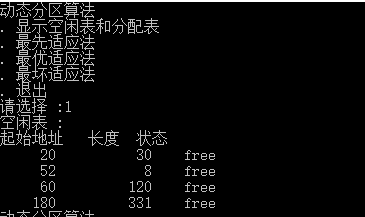最先适应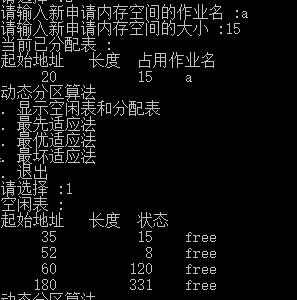最优适应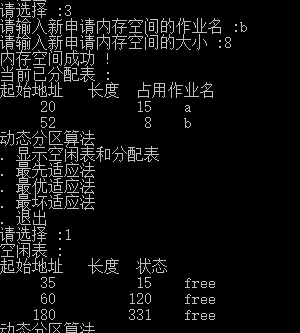最坏适应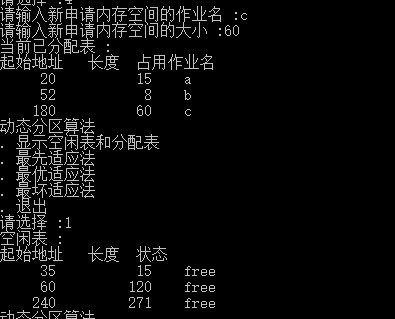展开全文c语言
• 常用的放置策略：首次匹配(首次适应算法)最佳匹配(最佳适应算法)最坏匹配(最坏适应算法) 一、首次适应算法（First Fit）：该算法从空闲分区链首开始查找，直至找到一个能满足其大小要求的空闲分区为止。然后再按照...
所谓动态分区分配 就是在处理作业的过程中，建立分区，依用户请求的大小分配分区。在分区回收的过程中会涉及一个空间利用效率相关的放置策略，即选择空闲区的策略。常用的放置策略：首次匹配(首次适应算法)最佳匹配(最佳适应算法)最坏匹配(最坏适应算法)      一、首次适应算法（First Fit）：该算法从空闲分区链首开始查找，直至找到一个能满足其大小要求的空闲分区为止。然后再按照作业的大小，从该分区中划出一块内存分配给请求者，余下的空闲分区仍留在空闲分区链    中。     特点： 该算法倾向于使用内存中低地址部分的空闲区，在高地址部分的空闲区很少被利用，从而保留了高地址部分的大空闲区。显然为以后到达的大作业分配大的内存空间创造了条件。     缺点：低地址部分不断被划分，留下许多难以利用、很小的空闲区，而每次查找又都从低地址部分开始，会增加查找的开销。       二、最佳适应算法（Best Fit）：该算法总是把既能满足要求，又是最小的空闲分区分配给作业。为了加速查找，该算法要求将所有的空闲区按其大小排序后，以递增顺序形成一个空白链。这样每次找到的第一个满足要求的空闲区，必然是最优的。孤立地看，该算法似乎是最优的，但事实上并不一定。因为每次分配后剩余的空间一定是最小的，在存储器中将留下许多难以利用的小空闲区。同时每次分配后必须重新排序，这也带来了一定的开销。     特点：每次分配给文件的都是最合适该文件大小的分区。     缺点：内存中留下许多难以利用的小的空闲区。      三、最坏适应算法（Worst Fit）：最坏适应算法是将输入的作业放置到主存中与它所需大小差距最大的空闲区中。空闲区大小由大到小排序。      特点：尽可能地利用存储器中大的空闲区。      缺点：绝大多数时候都会造成资源的严重浪费甚至是完全无法实现分配。而在实现过程中，首次适应算法与最佳适应算法的区别在于：首次适应算法中空闲区的排列顺序是按地址大小排列，小地址在前，大地址在后；而最佳适应算法中空闲区的队列排列是空闲区块的大小，小的在前，大的在后。我在实现时用了一个结构体来存储空闲区的信息，然后再用双链表来实现空闲区队列（我本来是准备用单链表的，但是在遇到需要将空闲区块前插到链表中时不太方便，然后就换成双链表结果了）。其次，空闲区合并问题有些许复杂，我一开始写了之后，运行发现出现上下两个都是空闲区时，程序并没有合并成功，而是只改变了空闲区块内的状态字，后来发现是条件的判断和退出出了问题。具体操作详见代码：#include <iostream>
using namespace std;
const int Max_length=640;//最大内存
int a, b;//a记录算法编号，b记录操作编号
struct freearea//空闲区的结构体
{
int ID;//分区号
int size;//分区大小
int address;//分区地址
bool flag;//使用状态，0为未占用，1为已占用
};
typedef struct DuNode//双向链表结点
{
freearea data;//数据域
DuNode *prior;//指针域
DuNode *next;
}*DuLinkList;
DuLinkList m_rid = new DuNode, m_last = new DuNode;//双向链表首尾指针
void init()//空闲区队列初始化
{
m_rid->prior = NULL;
m_rid->next = m_last;
m_last->prior = m_rid;
m_last->next = NULL;
m_rid->data.size = 0;
m_last->data.address = 0;
m_last->data.size = Max_length;
m_last->data.ID = 0;
m_last->data.flag = 0;//!!!
}
void show()
{
DuNode *p = m_rid->next;//指向空闲区队列的首地址
cout << "+++++++++++++++++++++++++++++++++++++++" << endl;
while (p)
{
cout << "分区号：   ";
if (p->data.ID == 0)
cout << "FREE" << endl;
else
cout << p->data.ID << endl;
cout << "分区起始地址：   " << p->data.address << endl;
cout << "分区大小：   " << p->data.size << endl;
cout << "分区状态：   ";
if (p->data.flag)
cout << "已被占用" << endl;
else
cout << "空闲" << endl;
cout << "——————————————————" << endl;
p = p->next;
}
}
bool first_fit(int id,int m_size)//首次适应算法
{
DuNode *p = m_rid->next;//指向空闲区队列的首地址
DuLinkList fa = new DuNode;//新建一个空闲区并赋值
//memset(fa, 0, sizeof(DuNode));
fa->data.ID = id;
fa->data.size = m_size;
fa->data.flag = 1;//表示被占用
while (p)//在空闲区列表中从低地址向高地址查找
{
if (p->data.size >= m_size && !p->data.flag)//找到大于等于所需的内存的空闲区
{
if (p->data.size == m_size)//刚好空闲区大小与所需大小相等
{
p->data.ID = id;
p->data.flag = 1;
free(fa);//清空新创建的空闲区
return true;
}
else if(p->data.size>m_size)//空闲区比所需内存大，则需要将多的内存作回收处理
{
fa->data.address = p->data.address;//将空闲区的前半部分分出去
p->data.address += m_size;
p->data.size -= m_size;
p->prior->next = fa;
fa->next = p;
fa->prior = p->prior;
p->prior = fa;
return true;
}
}
p = p->next;
}
free(fa);//查找分配失败
return false;
}
bool best_fit(int id, int m_size)//最佳适应算法，其中需要查找最佳的存放位置
{
DuNode *p = m_rid->next;//指向空闲区队列的首地址
DuNode *q = NULL;//存放最佳地址
DuLinkList fa = new DuNode;//新建一个空闲区并赋值
memset(fa, 0, sizeof(DuNode));
fa->data.ID = id;
fa->data.size = m_size;
fa->data.flag = 1;//表示被占用
int d = Max_length;//所需内存大小与空闲区大小之间的差值
while (p)//循环查找最小的差值d并记录此时的地址值
{
if (p->data.size >= m_size && !p->data.flag)//找到大于等于所需的内存的空闲区
{
if (p->data.size - m_size < d)
{
q = p;
d = q->data.size - m_size;
}
}
p = p->next;
}
if (q == NULL)//查找分配失败
return false;
else
{
if (d == 0)//刚好找到与所需内存大小一样的空闲区
{
p->data.ID = id;
p->data.flag = 1;
free(fa);//清空新创建的空闲区
return true;
}
else//空闲区比所需内存大，则需要将多的内存作回收处理
{
fa->data.address = q->data.address;//将空闲区的前半部分分出去
q->data.address += m_size;
q->data.size -= m_size;
q->prior->next = fa;
fa->next = q;
q->prior = fa;
fa->prior = q->prior;
return true;
}
}
}
bool allocation()//分配资源
{
int id, m_size;//输入的作业号和内存块大小
cout << "请输入作业编号（分区号）和申请的内存大小（KB）：";
cin >> id >> m_size;
if (m_size <= 0)//申请的内存大小小于等于0，申请失败
{
cout << "申请的内存大小错误！请重新输入" << endl;
return false;
}
if (a == 1)//首次适应算法
{
if (first_fit(id, m_size))
{
cout << "内存分配成功！" << endl;
show();//显示内存分布
}
else
cout << "内存不足，分配失败！" << endl;
return true;
}
if (a == 2)//最佳适应算法
{
if (best_fit(id, m_size))
{
cout << "内存分配成功！" << endl;
show();//显示内存分布
}
else
cout << "内存不足，分配失败！" << endl;
return true;
}
}
void recycle()//回收内存
{
int id;
cout << "请输入想要释放的作业号：";
cin >> id;
DuNode *p = m_rid->next;//指向空闲区队列的首地址
DuNode *p1 = NULL;//辅助向量
while (p)//查找需要释放的作业的地址并进行合并工作
{
if (p->data.ID == id)
{
p->data.ID = 0;
p->data.flag = 0;
if (!p->prior->data.flag)//与前一个空闲区相邻，则合并
{
p->prior->data.size += p->data.size;
p->prior->next = p->next;
p->next->prior = p->next;
}
if (!p->next->data.flag)//与后一个空闲区相邻，则合并
{
p->data.size += p->next->data.size;
p->next->next->prior = p;
p->next = p->next->next;
}
break;
}
p = p->next;
}
show();
}
void main()
{
cout << "******************动态分区分配模拟******************" << endl;
cout << "**********1.首次适应算法	 2.最佳适应算法**********" << endl;
cout << "请输入要选择的分配算法：";
cin >> a;
init();//初始化内存块
while (a != 1 && a != 2)
{
cout << "输入错误！请重新输入：" << endl;
cin >> a;
}
while (1)
{
cout << "****************************************************" << endl;
cout << "**********1.申请内存   2.释放内存   3.退出**********" << endl;
cout << "请输入操作数：";
cin >> b;
switch (b)
{
case 1:
allocation();
break;
case 2:
recycle();
break;
case 3:
default:
cout << "动态分区分配算法结束，再会！" << endl;
exit(1);
break;
}
}
}运行结果：测试数据为：初始状态可用内存空间为640KB，请求序列：作业1申请130作业2申请60作业3申请100作业2释放60作业4申请200作业3释放100作业1释放130作业5申请140作业6申请60作业7申请50作业6释放60首次适应算法：（测试结果只显示了部分）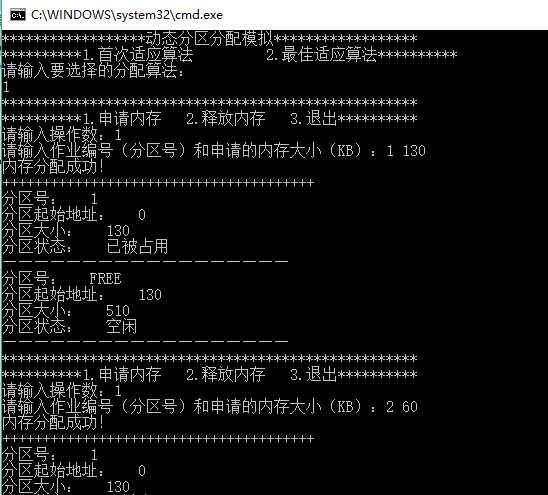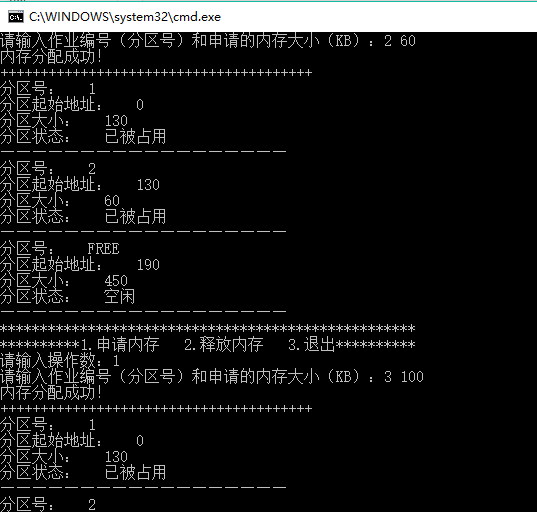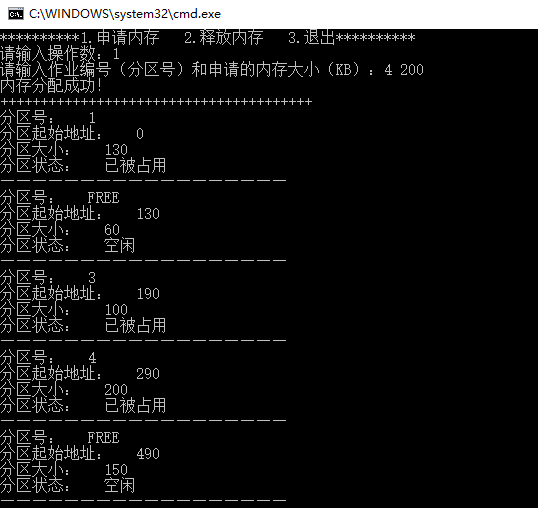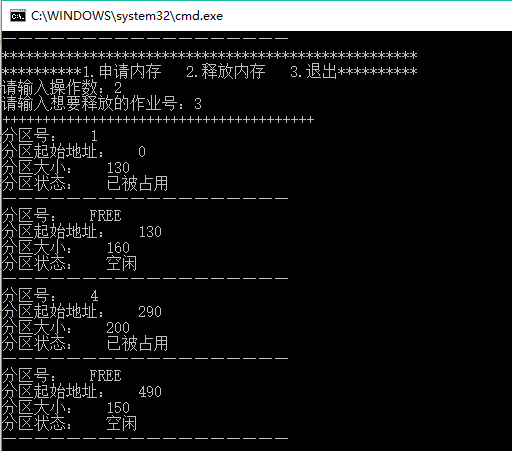最佳适应算法：（部分测试数据）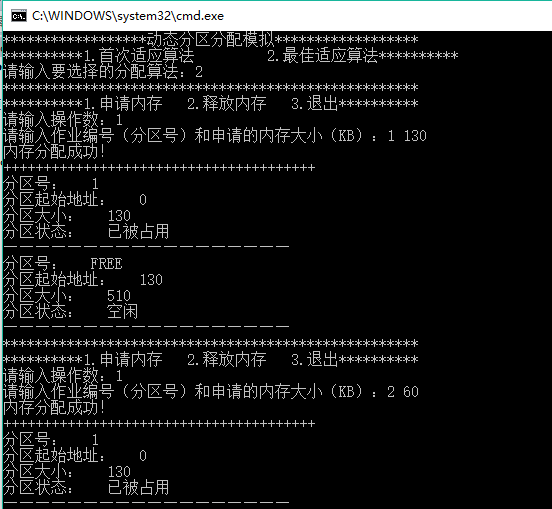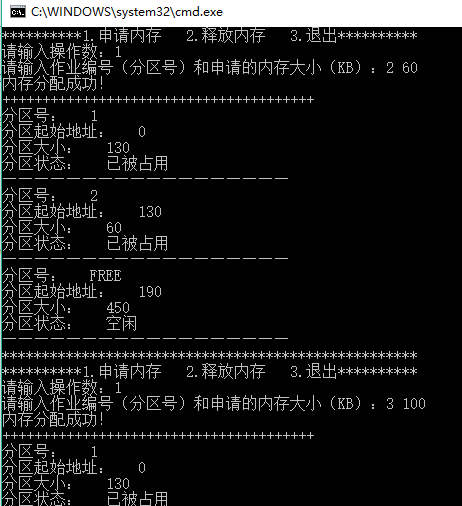展开全文• 大家好，我是一只学弱狗，记录学习的点点滴滴！ 优质文章 一张黄图的故事 JavaSE练习项目-坦克大战...基于顺序搜索的动态分区分配算法有如下四种：首次适应算法，循环首次适应算法、最佳适应算法和最坏适应算法。 首.

大家好，我是一只学弱狗，记录学习的点点滴滴！

优质文章

一张黄图的故事
JavaSE练习项目-坦克大战
我是菜鸟、我小试牛刀
linux指令太多记不住？小白看这篇就够了！

优质专栏

数据库就该这样学
爪哇外步篇

基于顺序搜索的动态分区分配算法
为了实现动态分区分配，通常是将系统中的空闲分区链接成一个链。所谓顺序搜索，是指依次搜索空闲分区链上的空闲分区，去寻找一个其大小能满足要求的分区。基于顺序搜索的动态分区分配算法有如下四种：首次适应算法，循环首次适应算法、最佳适应算法和最坏适应算法。
首次适应算法
算法思想
首次适应算法要求空闲分区链以地址递增的次序链接，在分配内存时，从链首开始查找，直到找到一个大小能满足要求的空闲分区为止。
优点
优先利用内存中低地址部分的空闲分区，从而保留了高地址部分的大空闲区，为大作业分配大的内存空间创造了条件。
缺点
低地址部分不断被划分，会留下许多难以利用的、很小的空闲分区。每次查找都是从低地址部分开始查找，这无疑怎能更加了查找可用空闲分区时的开销。
我的想法
参考循环首次适应算法，设置一个起始寻查指针，以指示下一次起始查寻的空闲分区，每次查找结束时，记录从开始位置到起始寻查指针位置之间的空闲分区的最大容量，当下一次查找时，如所需容量小于所记录的最大容量，则从开始位置开始查找，若所需容量大于所记录的最大容量，则从起始查寻指针的位置开始查找，从而减少了查看可用空闲分区时的开销。


展开全文操作系统
• 循环首次适应算法 最佳适应算法 最坏适应算法 内存中有0-100M的空间为用户程序空间，最开始用户空间是空闲的 作业数量、作业大小、进入内存时间、运行时间需要通过界面进行输入 可读取样例数据（要求存放在外部文件...
• 操作系统实验三，主存空间的分配与回收，包括首次适应算法、最佳和最坏适应算法，内含完整文档和源码，编译即可执行。
• 最佳适应算法（Best Fit） ...最坏适应算法（Worst Fit） 算法思想：与最佳适应算法刚好相反，将空闲分区链的分区按照从大到小的顺序排序形成空闲分区链，每次查找时只要看第一个空闲分区是否满足即可。
• 操作系统进程调度内存分配算法可视化模拟，java，idea 支持的算法： 先来先服务，时间片轮转，优先级调度 首次适应，最佳适应，最坏适应操作系统 内存分配
• 目录思维导图首次适应算法最佳适应算法最坏适应算法邻近适应算法总结 思维导图 首次适应算法 最佳适应算法 最坏适应算法 邻近适应算法 总结 注意: 一定要记住 首次适应 邻近适应 都是地址有关的。 最佳...操作系统
• 内含三个实验：进程调度（先来先服务、短进程优先）、作业调度（高优先级优先、轮转法、最高响应比优先）、内存块分配算法（首次适应、循环首次适应、最佳适应、最坏适应）。附加可执行exe文件导出的jar包。界面由...
• 实验四主存空间的分配和回收 ...采用连续分配方式之动态分区分配存储管理，使用首次适应算法、循环首次适应算法、最佳适应算法和最坏适应算法4种算法完成设计。 （1）**设计一个作业申请队列以及作业...
• - 动态分区分配算法 操作系统实验报告 实验四 动态分区分配算法 学号: 班级: 姓名: 实验目的 通过这次实验加深对动态分区分配算法的理解进一步掌握首次适应算法 循环首次适应算法最佳适应算法和最坏适应算法的实现...
• 实验四主存空间的分配和回收 ...采用连续分配方式之动态分区分配存储管理，使用首次适应算法、循环首次适应算法、最佳适应算法和最坏适应算法4种算法完成设计。 （1）**设计一个作业申请队列以及作业...
• 实验四主存空间的分配和回收 网络工程专业 姓名：蔡...采用连续分配方式之动态分区分配存储管理，使用首次适应算法、循环首次适应算法、最佳适应算法和最坏适应算法4种算法完成设计(任选两种算法)。 （1）设计一...
• 采用连续分配方式之动态分区分配存储管理，使用首次适应算法、循环首次适应算法、最佳适应算法和最坏适应算法4种算法完成设计。 （1）**设计一个作业申请队列以及作业完成后的释放顺序，实现...
• 操作系统原理课程设计：可变式分区存储管理系统 采用空闲区链实现主存的分配与回收 使用首次适应算法、最优适应算法、最坏适应算法、最后适应算法
• 实验四 主存空间的分配和回收 专业：商业软件工程 姓名：郭明茵 学号：201406114204 一、 实验目的 ...采用连续分配方式之动态分区分配存储管理，使用首次适应算法、循环首次适应算法、最佳适应算法和最坏...
• 实验四主存空间的分配和回收 ...采用连续分配方式之动态分区分配存储管理，使用首次适应算法、循环首次适应算法、最佳适应算法和最坏适应算法4种算法完成设计。 （1）**设计一个作业申请队列以及作业完成后的释......